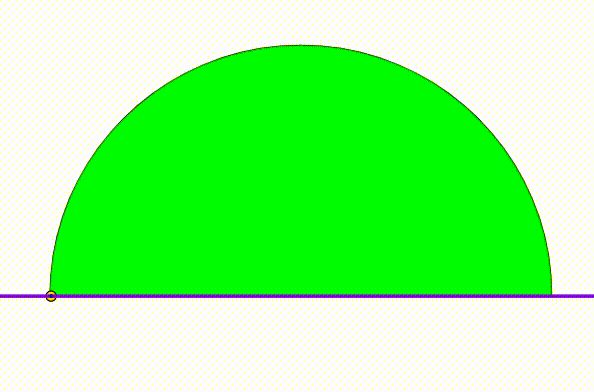# Dynamic Geometry: P134

Geometry Level 5The diagram shows a black semicircle with radius $1$. The cyan and green semicircles are tangent to each other and internally tangent to the black semicircle. They are growing and shrinking freely so that the sum of their radius is always equal to the black semicircle's radius. We draw a red vertical segment using their tangency point. At last we inscribed two yellow circles so they are tangent to the red line, to the black semicircle and to one of the two bottom semicircles. Using the tagency points, we draw a purple triangle and a blue triangle. We draw the Euler line of each triangle. When the Euler line are perpendicular, the radius of one yellow circle can be expressed as:

$\dfrac{p-q\sqrt{m}}{l}$

where $p$, $q$, $m$ and $l$ are relatively coprime positive integers. $m$ is square-free. Find $p+q+m+l$.

×